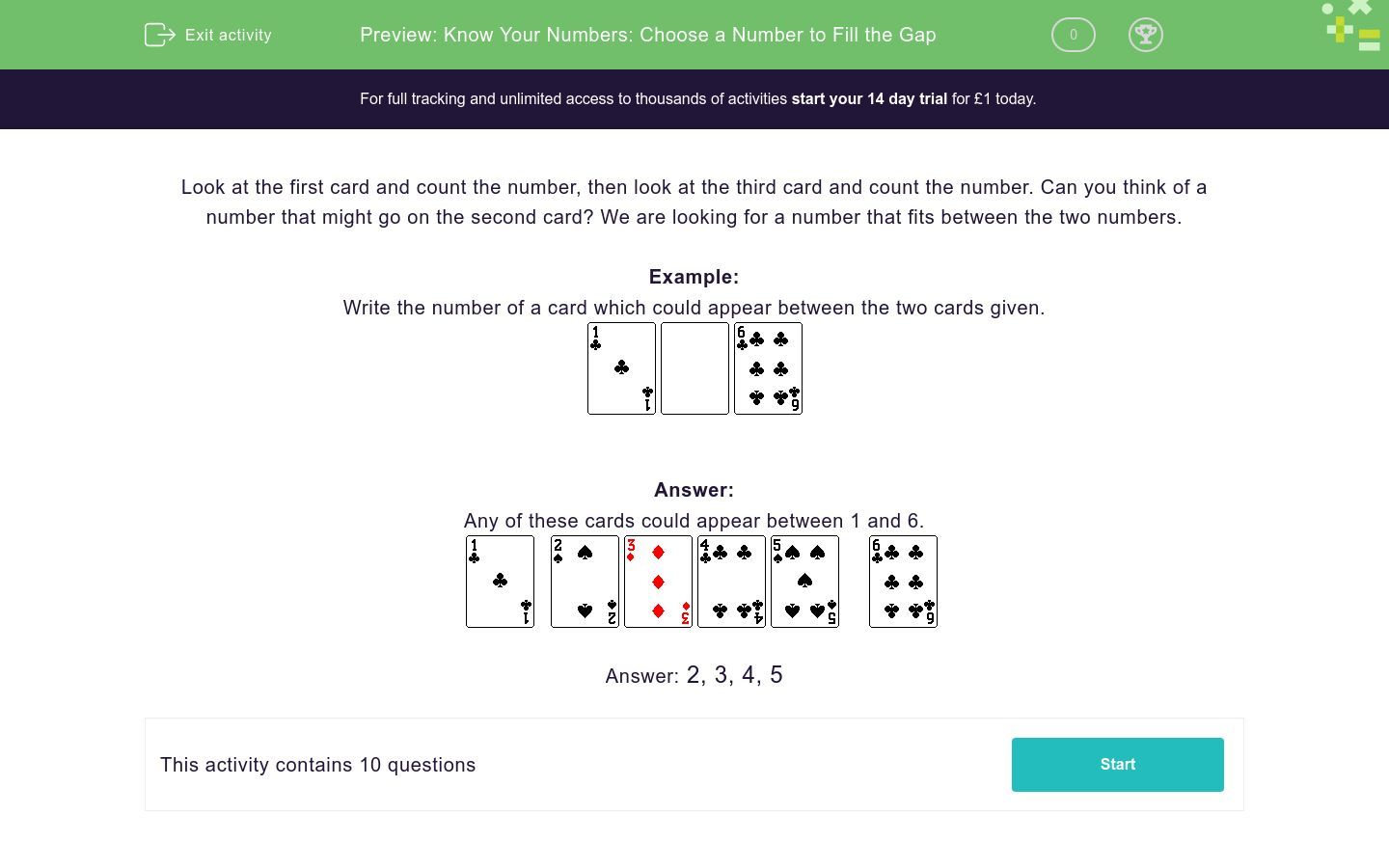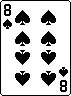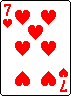# Know Your Numbers: Choose a Number to Fill the Gap

In this worksheet, students use their knowledge of number order to solve simple gap-fill problems in the range 0-20.Key stage:  KS 1

Curriculum topic:   Number: Number and Place Value

Curriculum subtopic:   Count to 100

Difficulty level:### QUESTION 1 of 10

Look at the first card and count the number, then look at the third card and count the number. Can you think of a number that might go on the second card? We are looking for a number that fits between the two numbers.

Example:

Write the number of a card which could appear between the two cards given.Any of these cards could appear between 1 and 6.Write any number which could appear between the two given numbers.Write any number which could appear between the two given numbers.Write any number which could appear between the two given numbers.Write any number which could appear between the two given numbers.Write any number which could appear between the two given numbers.Write any number which could appear between the two given numbers.Write any number which could appear between the two given numbers.Write any number which could appear between the two given numbers.Write any number which could appear between the two given numbers.Write any number which could appear between the two given numbers.• Question 1

Write any number which could appear between the two given numbers.5
EDDIE SAYS
In sequence the numbers are 4, 5, 6.
• Question 2

Write any number which could appear between the two given numbers.4
5
EDDIE SAYS
4 or 5 can come between 3 and 6.
• Question 3

Write any number which could appear between the two given numbers.7
EDDIE SAYS
In sequence the numbers are 6, 7, 8.
• Question 4

Write any number which could appear between the two given numbers.8
9
EDDIE SAYS
8 or 9 could appear between 7 and 10.
• Question 5

Write any number which could appear between the two given numbers.2
3
4
5
EDDIE SAYS
2, 3, 4 or 5 could appear between 1 and 6.
• Question 6

Write any number which could appear between the two given numbers.4
5
6
7
8
9
EDDIE SAYS
4, 5, 6, 7, 8 or 9 could appear between 3 and 10.
• Question 7

Write any number which could appear between the two given numbers.2
3
EDDIE SAYS
2 or 3 could appear between 1 and 4.
• Question 8

Write any number which could appear between the two given numbers.7
8
9
EDDIE SAYS
7, 8 or 9 could appear between 6 and 10.
• Question 9

Write any number which could appear between the two given numbers.3
4
5
6
7
8
EDDIE SAYS
3, 4, 5, 6, 7 or 8 could appear between 2 and 9.
• Question 10

Write any number which could appear between the two given numbers.6
7
EDDIE SAYS
6 or 7 could appear between 5 and 8.
---- OR ----

Sign up for a £1 trial so you can track and measure your child's progress on this activity.

### What is EdPlace?

We're your National Curriculum aligned online education content provider helping each child succeed in English, maths and science from year 1 to GCSE. With an EdPlace account you’ll be able to track and measure progress, helping each child achieve their best. We build confidence and attainment by personalising each child’s learning at a level that suits them.

Get started